Previous: 7.2.2 Open System Up: 7.2 The Open Von Next: 7.2.4 A Brute Force

## 7.2.3 Self Consistency

The interactions between electrons involving distances greater than the Debye length can be treated using the Poisson equation.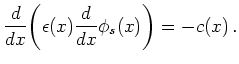(7.17)

Here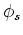denotes the (electrostatic) potential and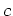denotes the total charge density (sum of doping and carrier charges). We use Dirichlet boundary conditions for the Poisson equation. The difference between left and right potential is equal to the bias. Alternatively it can be supposed that part of the voltage drop is in the electrodes. Such boundary conditions are for instance implemented in the program package NanoMOS [RVG+03].

Self-consistency in electronic device simulation means ensuring that the carrier density profile in the simulated device is consistent with its potential profile. The self-consistent field effectively acts to screen the positive applied voltage. Therefore, the effects of the self-consistent field can essentially be modeled by a smaller applied bias.

The Poisson and the von Neumann equation form a system of coupled nonlinear differential equations which is usually solved by an iteration between Poisson's and von Neumann's equation.

The potential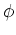which enters the Schrödinger equation (see Figure 7.1) is the sum of the solutionof the Poisson equation and anfrom the materials' bandgap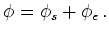(7.18)

The potential energy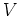in the Schrödinger equation is then given by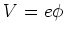(7.19)

where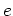is the carrier charge.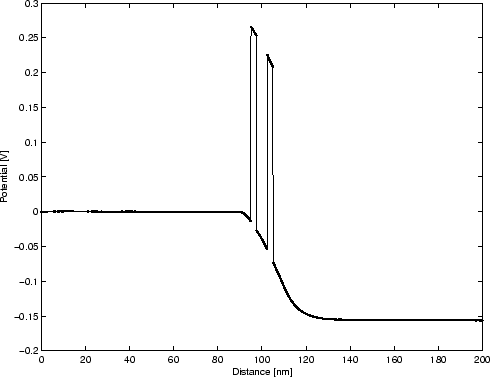In order to solve the coupled system, we have used the Gummel method [Gum64], which takes into account the exponential dependence of the density on the potential. We follow the approach from [BAPGR02].

With this the step for updating the potential in the iteration is given by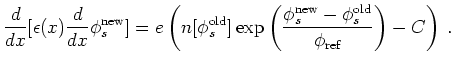(7.20)

Further details can be found in [BAPGR02], where this method, which is based on classical assumptions, was introduced.